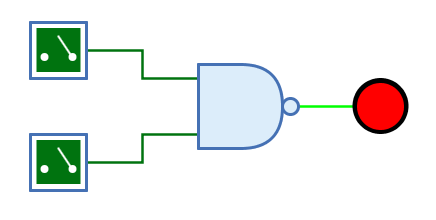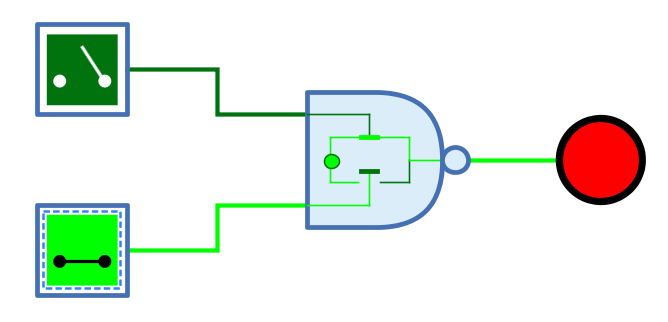## Appearance## Behavior

The NAND gate outputs the result of the NAND operation of the 1-bit signals at the input. The output has the value 0 only if all inputs have the value 1.

Table 1. Truth table
0 1 Z X

0

1

1

1

X

1

1

0

1

X

Z

1

1

1

X

X

X

X

X

X

(Z: Undefined/High impedance, X: Error)

The behaviour for unconnected inputs or input wires carrying an undefined signal can be changed using the system preference "Open Gate Input Behaviour".

The multi-bit version of the NAND gate will perform its one-bit transformation bitwise on its inputs.

### Mnemonics

The logical gates of Antares can illustrate their function with so-called "mnemonics". See the chapter Descriptions and explanations. Below is the mnemonic of the NAND gate.## Pins

Inputs

The 1-bit inputs of the NAND gate. Their number is determined by the attribute "Number of inputs".

Output

The 1-bit output of the NAND gate. Outputs the value of the calculated NAND function.

## Attributes

Orientation

The direction in which the output points.

Number of inputs

Determines how many inputs the NAND has. There are 2 to max. 8 inputs available. Can even be changed if the gate is already connected to wires.

Negate input n

When selected, the n-th input pin is negated. The maximum of "n" is determined by attribute "Number of inputs". Negation is also applied to the truth table and the mnemonics displayed for the gate.

Output Name

The optional name displayed next to the output. This can be useful when the NAND gate forms the end of a complex combinatorial circuit and the logical expression produced by the NAND gate needs to be specified.